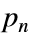# Problem 46057. Find the last non-zero digit in a primorial

Cody Problem 45251 asked us to find the last non-zero digit in a factorial. For this problem consider the primorial#, the product of primes up to and including the nth prime. For example, for n = 12, the primorial is 7420738134810, whose last non-zero digit is 1.
Find the last non-zero digit in the primorial#.
See also Cody Problems 46054 "Count trailing zeros in a primorial" and 46060 "Identify primorial primes".

### Solution Stats

29.51% Correct | 70.49% Incorrect
Last Solution submitted on Mar 31, 2023

### Community Treasure Hunt

Find the treasures in MATLAB Central and discover how the community can help you!

Start Hunting!